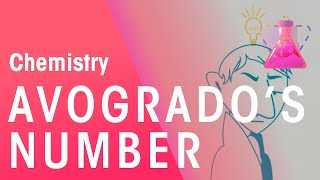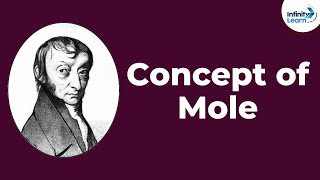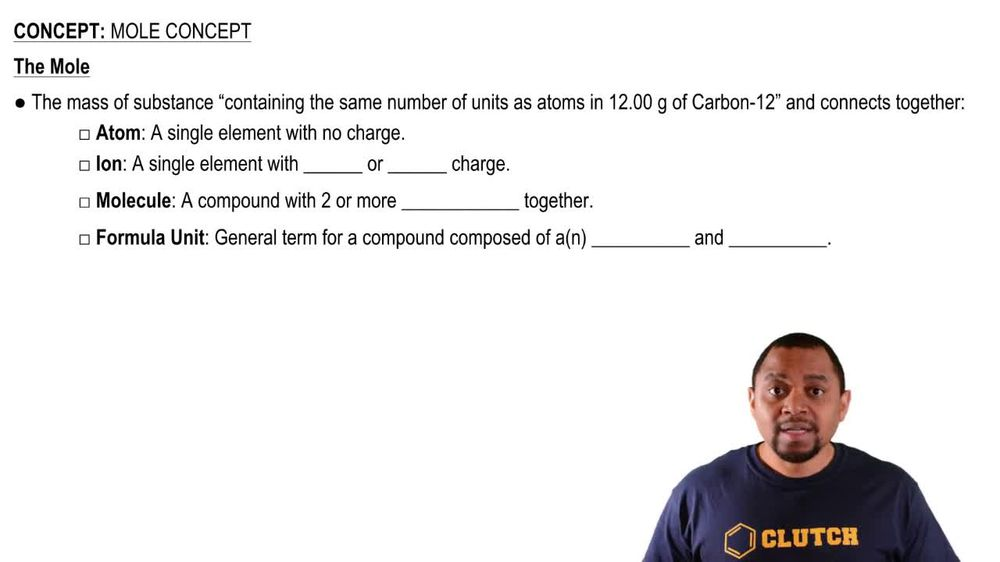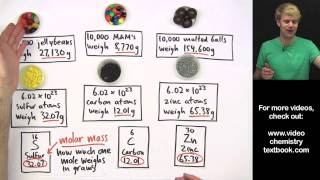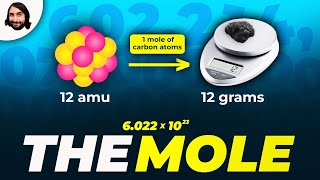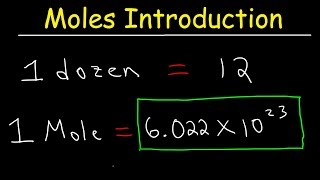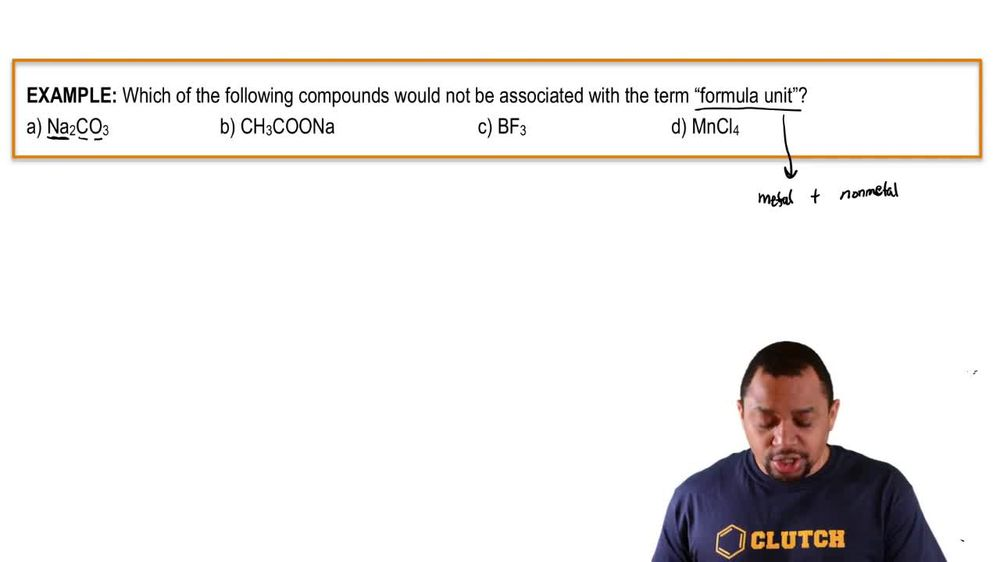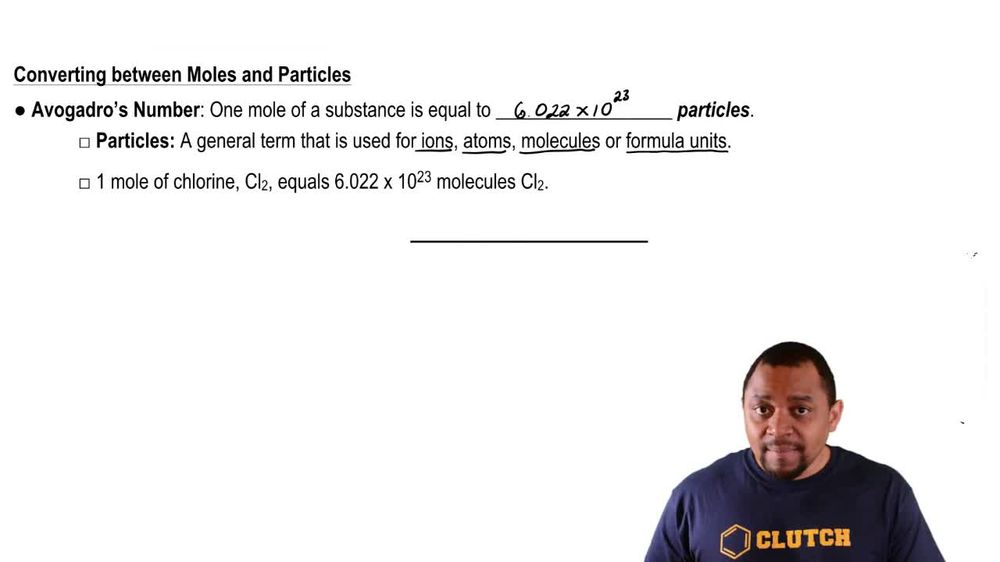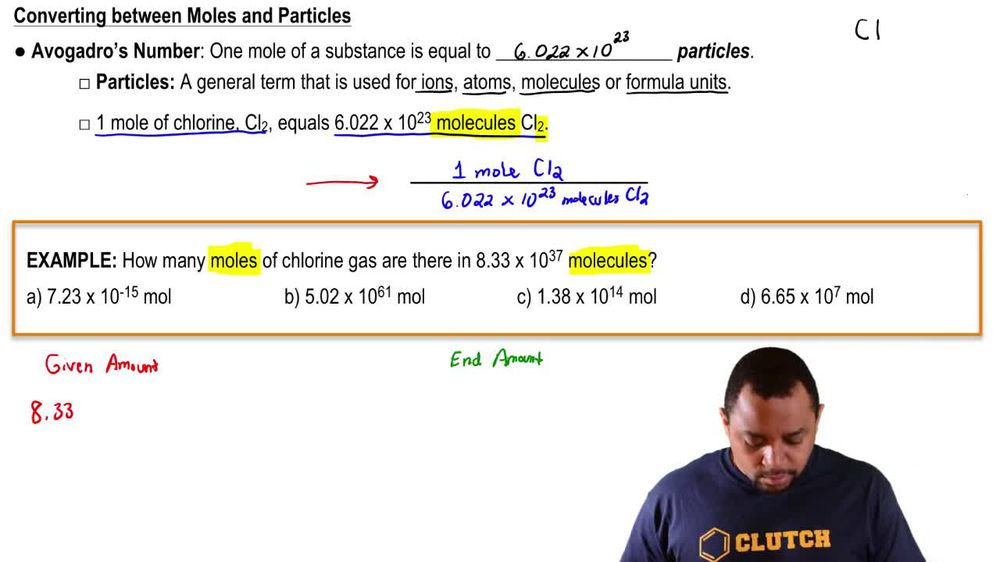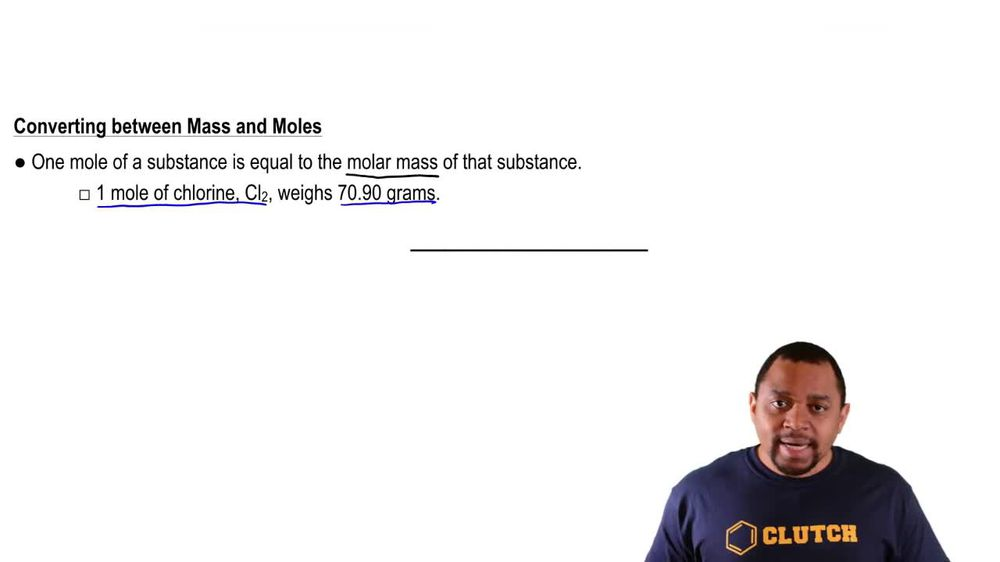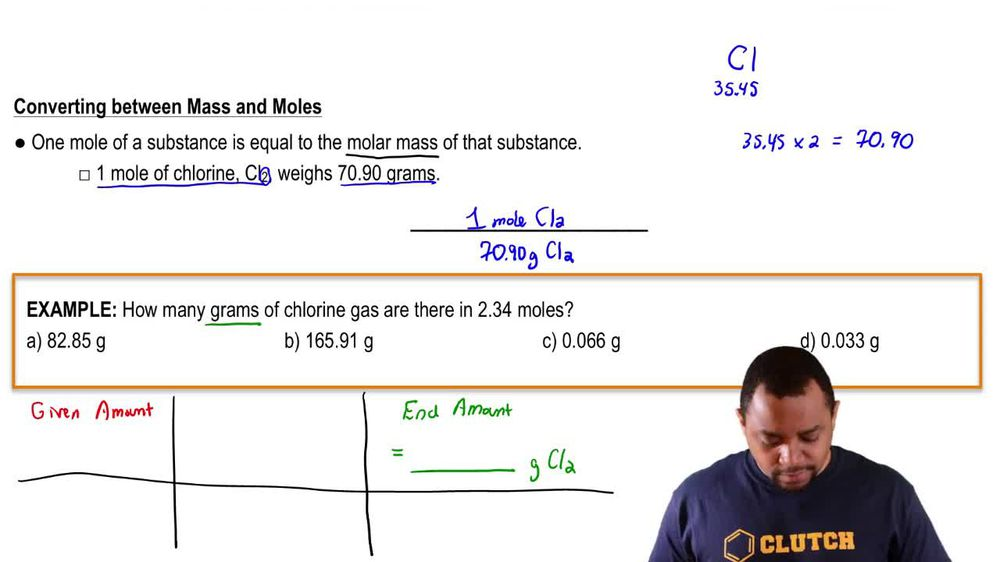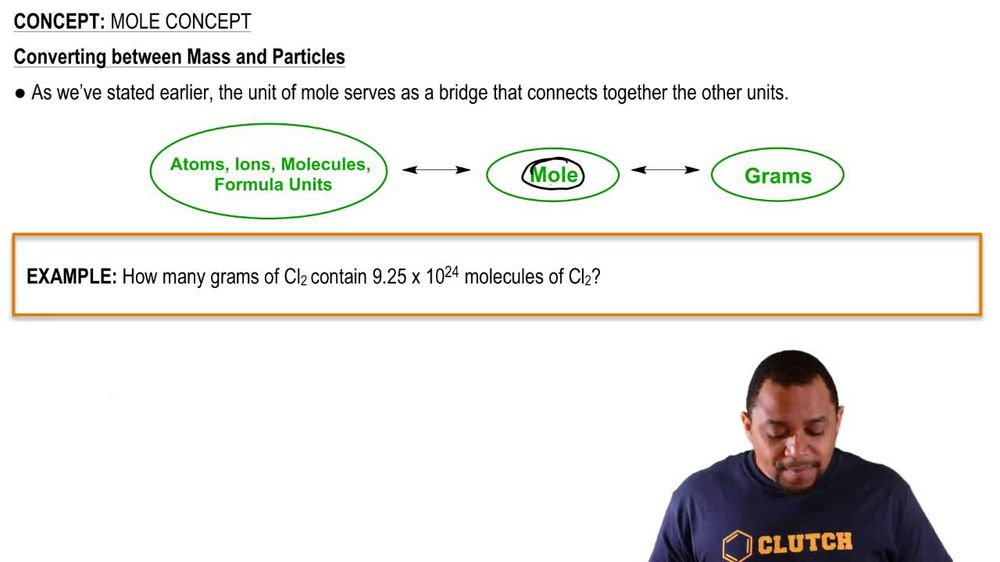Start typing, then use the up and down arrows to select an option from the list.
1. 2. Atoms & Elements2. Mole Concept
Problem

# Determine the number of moles of hydrogen atoms in each sample. c. 2.4 mol C6H12

Relevant Solution44s
Play a video:
Hey, everyone were asked how many moles of hydrogen, 0.0748 mole of octane half. So first, we're going to start off with our 0.0748 mall of octane And looking at our formula here, we can see that one mole of octane consists of 18 moles of hydrogen. So calculating this out, We end up with a value of 1.35 mole of hydrogen, which is our final answer. So I hope this made sense and let us know if you have any questions.